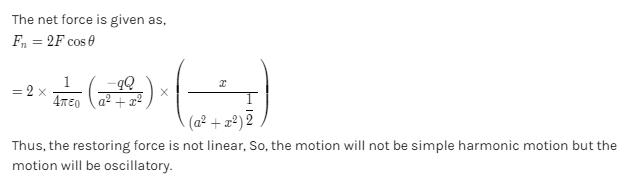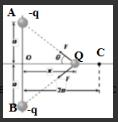Courses

# Electric Field And Potential MCQ Level - 2

## 10 Questions MCQ Test Basic Physics for IIT JAM | Electric Field And Potential MCQ Level - 2

Description
This mock test of Electric Field And Potential MCQ Level - 2 for Physics helps you for every Physics entrance exam. This contains 10 Multiple Choice Questions for Physics Electric Field And Potential MCQ Level - 2 (mcq) to study with solutions a complete question bank. The solved questions answers in this Electric Field And Potential MCQ Level - 2 quiz give you a good mix of easy questions and tough questions. Physics students definitely take this Electric Field And Potential MCQ Level - 2 exercise for a better result in the exam. You can find other Electric Field And Potential MCQ Level - 2 extra questions, long questions & short questions for Physics on EduRev as well by searching above.
QUESTION: 1

### Two point charges placed at a distance r in air experience a certain force. Then the distance at which they will experience the same force in a medium of dielectric constant k is

Solution: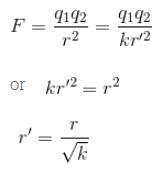The correct answer is: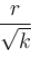QUESTION: 2

### Two isolated, charged conducting spheres of radii a and b produce the same electric field near their surfaces. The ratio of electric potentials on their surfaces is

Solution: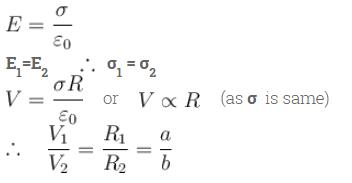QUESTION: 3

### Twenty seven drops of water of the same size are equally and similarly charged. They are then united  to from a bigger drop. By what factor will the electrical potential changes.

Solution: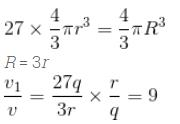The correct answer is: 9 times

QUESTION: 4

Electric potential at any point x, y, z in the space is given V=4x2−3x

Solution:

V = 4x2 - 3x
Ex = ∂V/∂x = 8x - 3
Ey = ∂V/∂y = 0
Ez = ∂V/∂z = 0
So, E = -(8x - 3)

QUESTION: 5

An electric dipole consisting of two opposite charge of 2 × 10–6C each separated by a distance of 0.03m is placed in an electric field of 2 × 105NC–1. The maximum torque on the dipole will be

Solution:

q = 2 × 10–6 C
2l = 0.03 m
E = 2 × 10
5 NC–1
τ = ρE sin θ Where p is the dipole moment.

For maximum torque,  sinθ = 1  and    τ = ρE
Max. Torque = q × 2l × E = 2 × 10–6 × 0.03 × 2 × 105 Nm
=
12 × 10–3 Nm
The correct answer is: 12 × 10–3Nm.

QUESTION: 6

Two identical point charge are placed at a separation of rP is a point on the line joining the charges, at a distance x from any one charge. The field at P is EE is plotted against x, for value of x from close to zero to slightly less than r. Which of the following best represent the resulting curve.

Solution: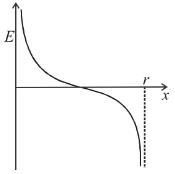QUESTION: 7

A spherical charged conductor has surface density σ of charge. The electric field on its surface is E. If the radius of the sphere is doubled keeping the surface density of charged unchanged then the electric field on the surface of the new sphere will be

Solution:

QUESTION: 8

A charge q is divided into two parts q1 and (q – q1). What is the ratio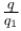so that the force between two parts placed at a given distance apart is maximum.

Solution:

F = k(q1)(q – q1)
F will be maximum. If q1(q – q1) is maximum
∴   q= (q – q1)
⇒ q = 2q1
⇒  q/q1 = 2:1
The correct answer is: 2 : 1

QUESTION: 9

Two concentric thin metallic sphere of radii R1 and (R1 > R2) carry charge Q1 and Q2 respectively. The potential at point P (distance from common centre is r) is : (Given : R1 < r < R2)

Solution:

The observation point is at a distance r from the centre of the inner sphere of radius R2. But it is inside the sphere of radius R1.

The correct answer is: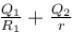QUESTION: 10

Two equal negative charge (q each) are fixed at point (0, a) and (0, –a) on the y-axis. A positive charge Q is released from rest at the points (29, 0) on the x-axis is the charge Q will

Solution: